# Team:Harvard BioDesign/Model

Harvard BioDesign 2016

## Model

### Informing our Design: Modelling Michaelis-Menten Kinetics

As we were refining the design of our plastic-sensing device, we were having a difficult time accounting for the lag between plastic uptake and plastic degradation. Recall that plastic in our bioreactor needs to be broken down by PETase in order to produce the terephthalic acid necessary to power our sensor. Though PETase is the most efficient PET-degrading enzyme discovered to date,1 it by no means degrades plastic instantaneously. This means that our devices gives off a signal at a later time and later location from where plastic was originally collected.

The team decided to turn to modelling to find quantitative solutions to this design challenge. We realized that understanding degradation rates as determined by enzyme kinetics would allow us to adjust our reactor design to minimize the lag in signal.

The task required us to understand enzyme kinetics under two broad sets of circumstances:

• When the pieces of plastic were small enough in size so that they were effectively suspended in a solution.
• When the pieces of plastic were too large to be considered in suspension. This would require us to apply models geared towards heterogeneous reactions that involve interactions between a solid substrate and an enzyme in solution.

To understand conditions involving small pieces of plastic, our team relied on Michaelis-Menten, a widely-applied model for enzyme kinetics. Prior to beginning our work, we ensured that the assumptions inherent in the model were reasonable for modelling PETase activity:2

• The model assumes that the product is not converted back into the substrate. This assumption holds when the concentration of product is very low. It is valid at the beginning of reactions and is especially compatible with our reactor design considering that the products of the reaction are funneled to another chamber where none of the original substrate or enzyme are present. The assumption also makes sense considering that our enzyme functions by breaking down polymers: after the reaction, the newly formed monomers no longer fit in the enzyme's active site.
• Another assumption is that the enzyme must be either free or bound to the substrate. That is, PETase should not at any time be bound to a molecule that does not consist of PET. The pnpb assays of Yoshida et al. (2016)1 during their characterization of different esterases demonstrate how PETase is significantly less likely that other cutinases to catalyze the breakdown of esters. This suggests that PETase has a more specific binding site and comes closer than alternative enzymes to satisfying the assumption.

An overview of Michaelis-Menten:3 The Michaelis-Menten constant (Km) corresponds to the substrate concentration at which the speed of the reaction is half of the maximum possible velocity: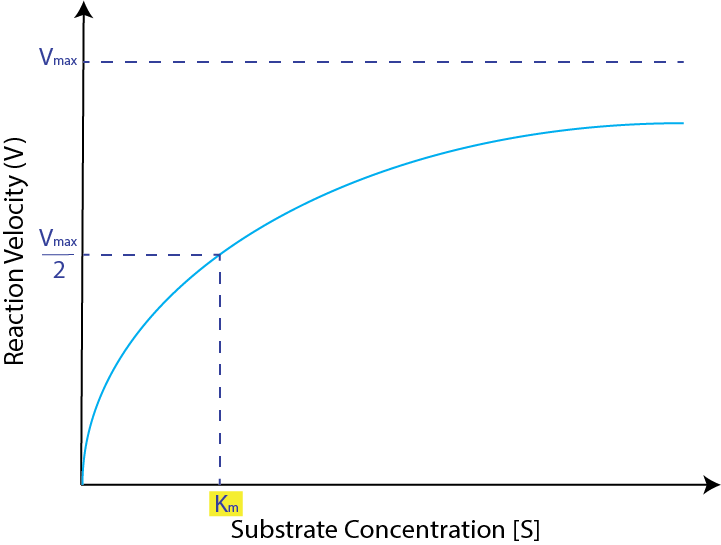Our team initially intended to compare the Michaelis-Menten constant associated with small plastics suspended in solutions to that of large pieces of plastics. However, we soon realized that the Michaelis-Menten model, with its references to substrate concentration, assumes that the substrate is in solution and would therefore be unsuitable for describing the kinetics associated with large solid substrates.

As our team and mentors investigated more sophisticated variations of Michaelis-Menten, we uncovered a highly relevant paper, "Surface Enzyme Kinetics for Biopolymer Microarrays: a Combination of Langmuir and Michaelis-Menten Concepts"4. This model incorporates additional parameters - adsorption and desorption coefficients - to help explain surface kinetics. Using the paper as a blueprint, our team constructed a model in Matlab (code available at the bottom of this page) that enables its users to enter values for adsorption, desorption, and catalysis constants and values for initial substrate and enzyme concentrations to immediately receive values for the Michaelis-Menten constant and the percentage of substrate that is bound to the enzyme at any point in time.

Since our assessment of enzyme kinetics was purely comparative (that is, we judged the efficiency of surface kinetics by comparing them to kinetics in solution), we focused our investigation on the parameters that are unique to surfaces: adsorption and desorption.

### Supporting Future Experiments: Calculating Extinction Coefficients

During our wet lab work with PETase this summer, we managed to successfully isolate the enzyme. In future quantitative experiments involving PETase, it would be useful to have a means by which we could calculate the concentration of this enzyme in a given solution. Although we have the necessary tools to measure the optical density (OD) of a solution that contains PETase, OD measurements are not sufficient on their own to determine concentration because we do not know how much light the enzyme absorbs.

This is where modelling comes into the picture. In order to calculate the concentration of enzyme present, our team also needs to know PETase's extinction coefficient. The extinction coefficient of an enzyme describes how much light the enzyme absorbs at a given wavelength. The paper, "Calculation of Protein Extinction Coefficients from Amino Acid Sequence Data",5 guided us through the process of finding this crucial coefficient for a wavelength of 280 nm and allowed us to use the number of tryptophan, tyrosine, and cystine amino acids present in PETase to arrive at an extinction coefficient of 39170 M-1 cm-1.

Below is the equation from the paper that enabled us to determine the extinction coefficient: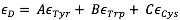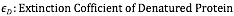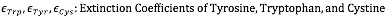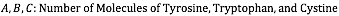Although this technically the coefficient for the denatured protein, the paper states explicitly that there is a "relatively small difference" between this coefficient and the coefficient of the native protein at 280 nm. It states that it is reasonably accurate to calculate it "directly... from amino acid composition information".

### MATLAB Code

%% PET Surface Enzyme Kinetics
% Define variables
h = 0.001; % step size
t = 1:h:100; % time frame
E = 0*t; %concentration of enzyme
k_cat = 0; % enzyme catalysis
k_a = 0; % enzyme adsorption
k_d = 0; % enzyme desorption
gamma_S = 0*t; % surface coverage of substrate
gamma_ES = 0*t; % surface coverage of enzyme-substrate complex
gamma_P = 0*t; % surface coverage of product
gamma_total = gamma_S + gamma_ES + gamma_P; % total number of surface sites
d_gamma_S = diff(gamma_S)/h % derivative of surface coverage of substrate wrt t
d_gamma_ES = diff(gamma_ES)/h % derivative of surface coverage of complex wrt t
d_gamma_P = diff(gamma_P)/h % derivative of surface coverage of product wrt t
theta_S = gamma_S/gamma_total; % relative surface coverage of substrate
theta_ES = gamma_ES/gamma_total; % relative surface coverage of complex
theta_P = gamma_P/gamma_total; % relative surface coverage of product
d_theta_S = diff(theta_S)/h % derivative of relative coverage of substrate wrt t
d_theta_ES = diff(theta_ES)/h % derivative of relative coverage of complex wrt t
d_theta_P = diff(theta_P)/h % derivative of relative coverage of product wrt t
% Set-up
d_gamma_ES = k_a*gamma_S - k_d*gamma_ES - k_cat*gamma_ES; % rxn rate for production of complex
d_gamma_P = k_cat*gamma_ES; % rxn rate for production of product
% Rates of change of surface coverage
d_theta_ES = k_a*theta_S - k_d*theta_ES - k_cat*theta_ES;
d_theta_P = k_cat*theta_ES;
% Assuming k_cat << k_a, k_d
theta_ES = k_a*theta_S*E/(k_d + k_cat); % equilibrium surface coverage of complex
K_m = k_d + k_cat/(k_a); % Michaelis-Menten constant

### Footnotes

1Yoshida, Shosuke et al. “A Bacterium That Degrades and Assimilates Poly(ethylene Terephthalate).” Science 351.6278 (2016): 1196–1199. science.sciencemag.org. Web.
2Montagud, A., E. Navarro, P. Córdoba, and J. Urchueguía. Kinetic Chemistry. N.p.: InterTech, n.d. Openwetware.org. Web. 6 July 2016.
3"Michaelis-Menten Equation - Interactive Graph." PhysiologyWeb. N.p., 1 Sept. 2014. Web. 14 July 2016.
4Lee, Hye, Alastair Wark, Terry Goodrich, Shiping Fang, and Robert Corn. "Surface Enzyme Kinetics for Biopolymer Microarrays: A Combination of Langmuir and Michaelis-Menten Concepts." American Chemical Society (2005): n. pag. Web. 26 July 2016. 5 Gill, Stanley, and Peter Hippel. "Calculation of Protein Extinction Coefficients from Amino Acid Sequence Data." Analytical Biochemistry (1989): n. pag. Web.